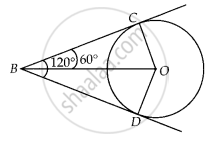# If from an external point B of a circle with centre O, two tangents BC and BD are drawn such that ∠DBC = 120°, prove that BC + BD = BO, i.e., BO = 2BC. - Mathematics

Diagram
Sum

If from an external point B of a circle with centre O, two tangents BC and BD are drawn such that ∠DBC = 120°, prove that BC + BD = BO, i.e., BO = 2BC.

#### SolutionTo prove: BO = 2BC

Given, ∠DBC = 120°

Join OC, OD and BO.

Since, BC and BD are tangents.

∴ OC ⊥ BC and OD ⊥ BD

We know, OB is the angle bisector of ∠DBC.

∴∠OBC = ∠DBO = 60°

In right-angled ∆OBC

cos 60^circ = (BC)/(OB)

⇒ 1/2 = (BC)/(OB)

⇒ OB = 2 BC

Also, BC = BD  ......[Tangent drawn from external point to circle are equal]

OB = BC + BC

⇒ OB = BC + BD

Concept: Number of Tangents from a Point on a Circle
Is there an error in this question or solution?

#### APPEARS IN

NCERT Mathematics Exemplar Class 10
Chapter 9 Circles
Exercise 9.3 | Q 3 | Page 107
Share